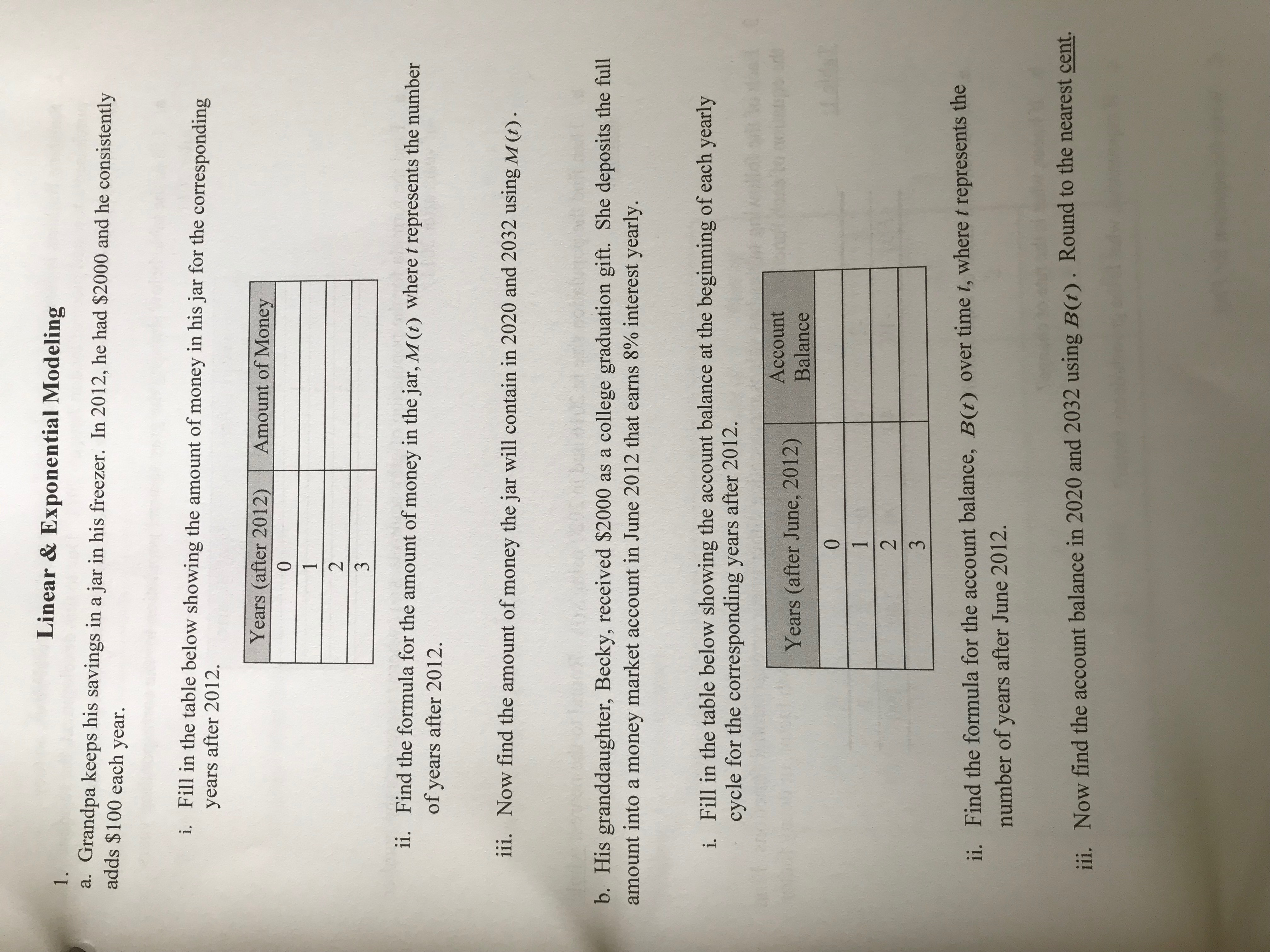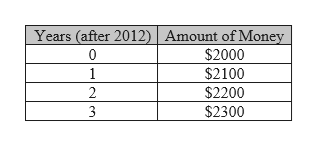Linear & Exponential Modeling1.Grandpa keeps his savings ina jar in his freezer. In 2012, he had \$2000 and he consistentlyadds \$100 each year.а.i. Fill in the table below showing the amount of money in his jar for the correspondingyears after 2012Years (after 2012)Amount of Money0123ii.Find the formula for the amount of money in the jar, M(t) where t represents the numberof years after 2012iii.Now find the amount of money the jar will contain in 2020 and 2032 using M (t)b. His granddaughter, Becky, received \$2000 as a college graduation gift. She deposits the fullamount into a money market account in June 2012 that earns 8% interest yearly.Fill in the table below showing the account balance at the beginning of each yearlycycle for the corresponding years after 2012.i.AccountYears (after June, 2012)Balance1t0123ii. Find the formula for the account balance, B(t) over time t, where t represents thenumber of years after June 2012.B(t). Round to the nearest cent.Now find the account balance in 2020 and 2032 usingiii.

Questionhelp_outlineImage TranscriptioncloseLinear & Exponential Modeling 1. Grandpa keeps his savings ina jar in his freezer. In 2012, he had \$2000 and he consistently adds \$100 each year. а. i. Fill in the table below showing the amount of money in his jar for the corresponding years after 2012 Years (after 2012) Amount of Money 0 1 2 3 ii. Find the formula for the amount of money in the jar, M(t) where t represents the number of years after 2012 iii. Now find the amount of money the jar will contain in 2020 and 2032 using M (t) b. His granddaughter, Becky, received \$2000 as a college graduation gift. She deposits the full amount into a money market account in June 2012 that earns 8% interest yearly. Fill in the table below showing the account balance at the beginning of each yearly cycle for the corresponding years after 2012. i. Account Years (after June, 2012) Balance 1t 0 1 2 3 ii. Find the formula for the account balance, B(t) over time t, where t represents the number of years after June 2012. B(t). Round to the nearest cent. Now find the account balance in 2020 and 2032 using iii. fullscreen
Step 1

Since we only answer up to 3 sub-parts, we’ll answer the first 3. Please resubmit the question and specify the other subparts (up to 3) you’d like answered

a.

Given grandpa keeps his savings in a jar in his freezer. In 2012, he had \$2000 and he consistently adds \$100 each year.

Step 2
1. The table showing the amount of the money in his jar for the corresponding years after 2012 is to be completed.

Since 0 represents the year 2012, the amount in the jar is \$2000.

So 1 represents the year 2013. Since \$100 is added to the jar each year, the amount in the jar is \$2000 + \$100 = \$2100.

Similarly 2 represents the year 2014 and the amount in the jar is \$2100 + \$100 = \$2200

And 3 represents the year 2015 and the amount in the jar is \$2200 + \$100 = \$2300.help_outlineImage TranscriptioncloseYears (after 2012) Amount of Money \$2000 \$2100 \$2200 \$2300 0 1 2 n fullscreen
Step 3
1. In 2012, the amount in the jar is \$2000.

For each year \$100 is added.

So for t years, the amount added would be given by (\$100)x...

Want to see the full answer?

See Solution

Want to see this answer and more?

Our solutions are written by experts, many with advanced degrees, and available 24/7

See Solution
Tagged in

Algebra